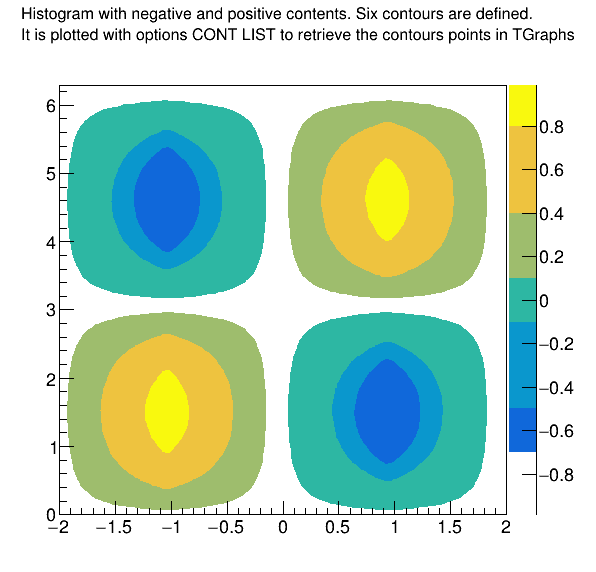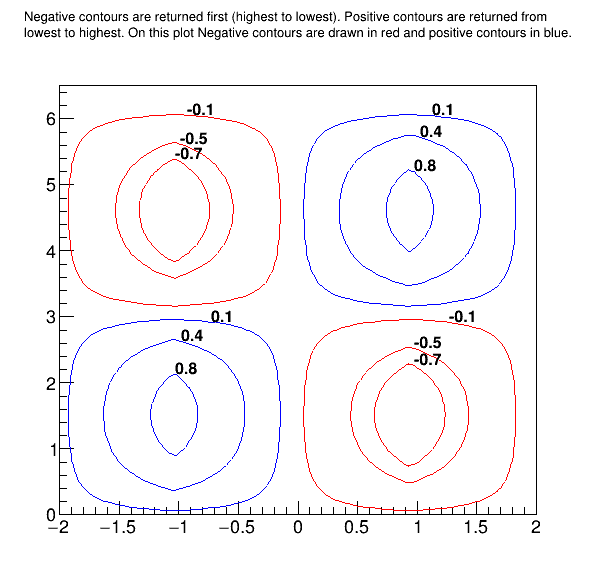# Contour List¶

Getting Contours From TH2D.

#### Image produced by .x ContourList.C¶

The contours values are drawn next to each contour.

#### Output produced by .x ContourList.C¶

It shows that 6 contours and 12 graphs were found.

#### ContourList.C¶

Author: Josh de Bever (CSI Medical Physics Group, The University of Western Ontario, London, Ontario, Canada), Olivier Couet
This notebook tutorial was automatically generated with ROOTBOOK-izer from the macro found in the ROOT repository on Thursday, May 19, 2022 at 08:10 AM.

In :
Double_t SawTooth(Double_t x, Double_t WaveLen);


A helper function is created:

In :
%%cpp -d
Double_t SawTooth(Double_t x, Double_t WaveLen){

// This function is specific to a sawtooth function with period
// WaveLen, symmetric about x = 0, and with amplitude = 1. Each segment
// is 1/4 of the wavelength.
//
//           |
//      /\   |
//     /  \  |
//    /    \ |
//   /      \
//  /--------\--------/------------
//           |\      /
//           | \    /
//           |  \  /
//           |   \/
//

Double_t y;
if ( (x < -WaveLen/2) || (x > WaveLen/2)) y = -99999999; // Error X out of bounds
if (x <= -WaveLen/4) {
y = x + 2.0;
} else if ((x > -WaveLen/4) && (x <= WaveLen/4)) {
y = -x ;
} else if (( x > WaveLen/4) && (x <= WaveLen/2)) {
y = x - 2.0;
}
return y;
}

In :
const Double_t PI = TMath::Pi();

TCanvas* c = new TCanvas("c","Contour List",0,0,600,600);
c->SetRightMargin(0.15);
c->SetTopMargin(0.15);

Int_t i, j;

Int_t nZsamples   = 80;
Int_t nPhiSamples = 80;

Double_t HofZwavelength = 4.0;       // 4 meters
Double_t dZ             =  HofZwavelength/(Double_t)(nZsamples - 1);
Double_t dPhi           = 2*PI/(Double_t)(nPhiSamples - 1);

TArrayD z(nZsamples);
TArrayD HofZ(nZsamples);
TArrayD phi(nPhiSamples);
TArrayD FofPhi(nPhiSamples);


Discretized z and phi values

In :
for ( i = 0; i < nZsamples; i++) {
z[i] = (i)*dZ - HofZwavelength/2.0;
HofZ[i] = SawTooth(z[i], HofZwavelength);
}

for(Int_t i=0; i < nPhiSamples; i++){
phi[i] = (i)*dPhi;
FofPhi[i] = sin(phi[i]);
}


Create histogram

In :
TH2D *HistStreamFn = new TH2D("HstreamFn",
"#splitline{Histogram with negative and positive contents. Six contours are defined.}{It is plotted with options CONT LIST to retrieve the contours points in TGraphs}",
nZsamples, z, z[nZsamples-1], nPhiSamples, phi, phi[nPhiSamples-1]);


In :
for (Int_t i = 0; i < nZsamples; i++) {
for(Int_t j = 0; j < nPhiSamples; j++){
HistStreamFn->SetBinContent(i,j, HofZ[i]*FofPhi[j]);
}
}

gStyle->SetOptStat(0);
gStyle->SetTitleW(0.99);
gStyle->SetTitleH(0.08);

Double_t contours;
contours = -0.7;
contours = -0.5;
contours = -0.1;
contours =  0.1;
contours =  0.4;
contours =  0.8;

HistStreamFn->SetContour(6, contours);


Draw contours as filled regions, and save points

In :
HistStreamFn->Draw("CONT Z LIST");
c->Update(); // Needed to force the plotting and retrieve the contours in TGraphs


Get contours

In :
TObjArray *conts = (TObjArray*)gROOT->GetListOfSpecials()->FindObject("contours");
TList* contLevel = NULL;
TGraph* curv     = NULL;
TGraph* gc       = NULL;

Int_t nGraphs    = 0;
Int_t TotalConts = 0;

if (conts == NULL){
printf("*** No Contours Were Extracted!\n");
TotalConts = 0;
return 0;
} else {
TotalConts = conts->GetSize();
}

printf("TotalConts = %d\n", TotalConts);

for(i = 0; i < TotalConts; i++){
contLevel = (TList*)conts->At(i);
printf("Contour %d has %d Graphs\n", i, contLevel->GetSize());
nGraphs += contLevel->GetSize();
}

nGraphs = 0;

TCanvas* c1 = new TCanvas("c1","Contour List",610,0,600,600);
c1->SetTopMargin(0.15);
TH2F *hr = new TH2F("hr",
"#splitline{Negative contours are returned first (highest to lowest). Positive contours are returned from}{lowest to highest. On this plot Negative contours are drawn in red and positive contours in blue.}",
2, -2, 2, 2, 0, 6.5);

hr->Draw();
Double_t xval0, yval0, zval0;
TLatex l;
l.SetTextSize(0.03);
char val;

for(i = 0; i < TotalConts; i++){
contLevel = (TList*)conts->At(i);
if (i<3) zval0 = contours[2-i];
else     zval0 = contours[i];
printf("Z-Level Passed in as:  Z = %f\n", zval0);

// Get first graph from list on curves on this level
curv = (TGraph*)contLevel->First();
for(j = 0; j < contLevel->GetSize(); j++){
curv->GetPoint(0, xval0, yval0);
if (zval0<0) curv->SetLineColor(kRed);
if (zval0>0) curv->SetLineColor(kBlue);
nGraphs ++;
printf("\tGraph: %d  -- %d Elements\n", nGraphs,curv->GetN());

// Draw clones of the graphs to avoid deletions in case the 1st
gc = (TGraph*)curv->Clone();
gc->Draw("C");

sprintf(val,"%g",zval0);
l.DrawLatex(xval0,yval0,val);
curv = (TGraph*)contLevel->After(curv); // Get Next graph
}
}
c1->Update();
printf("\n\n\tExtracted %d Contours and %d Graphs \n", TotalConts, nGraphs );
gStyle->SetTitleW(0.);
gStyle->SetTitleH(0.);
return c1;

TotalConts = 6
Contour 0 has 2 Graphs
Contour 1 has 2 Graphs
Contour 2 has 2 Graphs
Contour 3 has 2 Graphs
Contour 4 has 2 Graphs
Contour 5 has 2 Graphs
Z-Level Passed in as:  Z = -0.100000
Graph: 1  -- 147 Elements
Graph: 2  -- 147 Elements
Z-Level Passed in as:  Z = -0.500000
Graph: 3  -- 93 Elements
Graph: 4  -- 93 Elements
Z-Level Passed in as:  Z = -0.700000
Graph: 5  -- 65 Elements
Graph: 6  -- 65 Elements
Z-Level Passed in as:  Z = 0.100000
Graph: 7  -- 147 Elements
Graph: 8  -- 147 Elements
Z-Level Passed in as:  Z = 0.400000
Graph: 9  -- 107 Elements
Graph: 10  -- 107 Elements
Z-Level Passed in as:  Z = 0.800000
Graph: 11  -- 49 Elements
Graph: 12  -- 49 Elements

Extracted 6 Contours and 12 Graphs


Draw all canvases

In :
gROOT->GetListOfCanvases()->Draw()